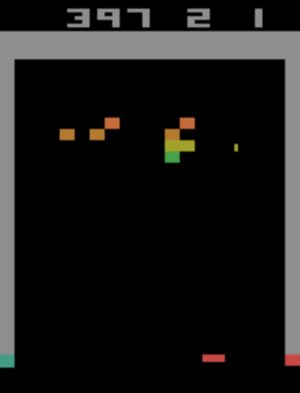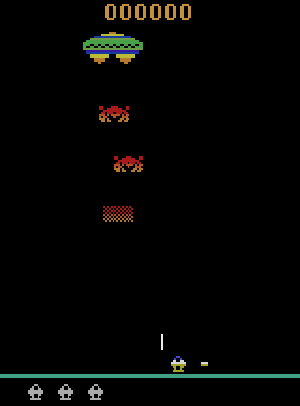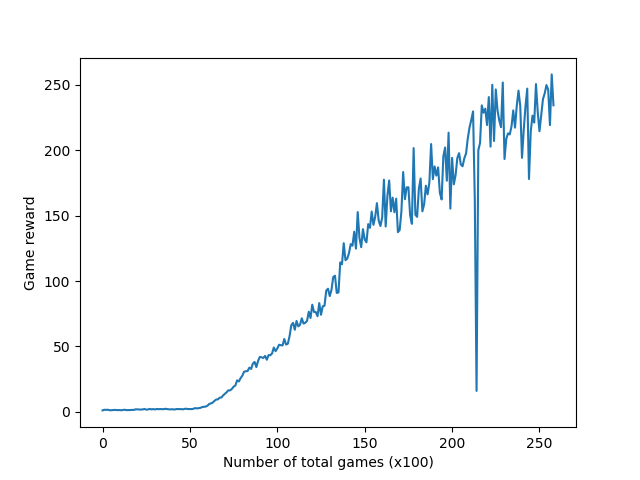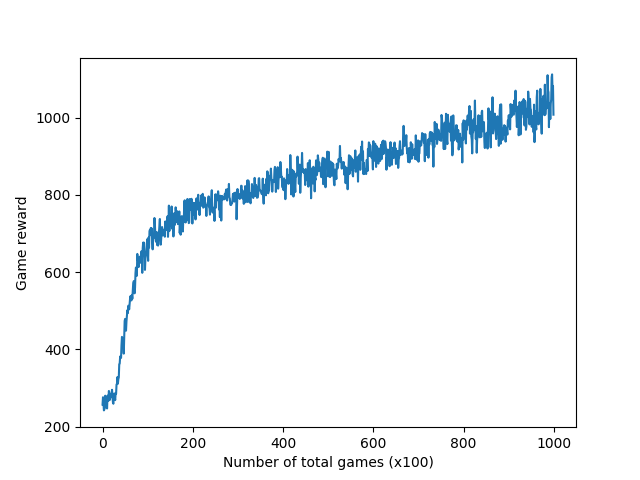# FLARE

## Design

FLARE is a reinforcement learning (RL) framework for training embodied agents with PyTorch. The design philosophy of FLARE is to maximize its flexibility so that a researcher can easily apply it to a variety of scenarios or tasks. By “easily”, we mean at least four properties:

1. The code is modular. For a new problem the researcher might only need to change some (hopefully a small number of) modules of the existing code while keeping the rest unchanged. For example, when the user decides to switch from “Q-learning” to “SARSA”, he/she probably only has to change the learning objective but not the network configuration (since both algorithms require outputting Q-values).

2. The code is extensible. Usually an embodied agent would have multiple tasks learned in parallel (e.g., language ability and vision ability). And sometimes some other unsupervised learning tasks might be added to facilitate feature learning. So FLARE should be able to easily combine several tasks that require distinct objective functions, or to easily add a new task to existing ones whenever the researcher feels necessary.

3. The support for complex computations due to the broad range of various algorithms that are involved in modeling an embodied agent. It should be convenient in FLARE to have computational branches of neural nets and conditional code behaviors. For this reason, we choose PyTorch because of its dynamic computational graph and that it blends well in the host language.

4. Extensible data I/O between an agent and different environments. In FLARE, the researcher only needs to spend a tiny effort on the change of agent-environment communication when the agent is placed in a new environment that has new sensor inputs or requires new actions.

## Prerequisites

FLARE is currently a pure Python framework which doesn’t need build. Several dependent tools:

That’s it. Enjoy training agents with FLARE!

## Add FLARE to python paths

To add the repository to your python paths permanently, append the following line to `~/.bashrc`:

``````export PYTHONPATH=<flare_root>:\$PYTHONPATH
``````

## A quick tutorial in five minutes

Some quick examples can be found in `flare/examples`. Below we show step-by-step code to train the “CartPole-v0” game from OpenAI Gym.

a) First we import different Python modules which all together constitute the overall training pipeline. Some modules such as `SimpleAC`, `SimpleModelAC`, and `SimpleRLAgent` were already implemented by us as examples of Algorithm, Model, and Agent classes. The users are encouraged to modify or write their own for more complex problems. This advanced topic of user customizing modules is explained in details in Modules.

``````"""
flare/examples/ac_example.py
"""

## import PyTorch nn module
import torch.nn as nn
## use a simple Actor-critic algorithm
from flare.algorithm_zoo.simple_algorithms import SimpleAC
## import the Manager to manage agents running in parallel
from flare.framework.manager import Manager
## use a simple network to compute the policy and value
from flare.model_zoo.simple_models import SimpleModelAC
## RL agent with simple control logic
from flare.agent_zoo.simple_rl_agents import SimpleRLAgent
## the agent collects data in an on-line manner without a replay buffer
from flare.framework.agent import OnlineHelper
## import a wrapped version of OpenAI Gym
from flare.framework.env import GymEnv
``````

b) Then we configure the problem and initialize the environments.

``````game = "CartPole-v0"

num_agents = 16    ## 16 agents running in parallel
num_games = 8000   ## each agent runs for 8000 games

env = GymEnv(game)
## get the shape of observation 'sensor'
state_shape = env.observation_dims()["sensor"]
## get the number of action 'action'
num_actions = env.action_dims()["action"]

# Spawn 16 agents and add an environment to each agent.
# Agent's behavior depends on the actual algorithm being used. Since we
# are using SimpleAC, a proper type of Agent is SimpleRLAgent.
reward_shaping_f = lambda x: x / 100.0 # when learning, we scale the reward by 0.01
agents = []
for _ in range(num_agents):
agent = SimpleRLAgent(num_games, reward_shaping_f=reward_shaping_f)
agent.set_env(GymEnv, game_name=game)
agents.append(agent)
``````

c) Next we construct the neural network and choose the algorithm to train the network.

``````## For this simple problem, our model uses an MLP to directly map
## each input vector to a latent embedding of 128 dimensions.
mlp = nn.Sequential(
nn.Linear(state_shape, 128),
nn.ReLU(),
nn.Linear(128, 128), nn.ReLU(), nn.Linear(128, 128), nn.ReLU())

## The learning algorithm is specified through defining an Algorithm object,
## which takes a Model object as its argument. In this example, we uses an
## algorithm called SimpleAC; it implements simple Actor-critic by taking
## the latent embedding output by the model defined above to generate a discrete
## policy and a value.
alg = SimpleAC(
model=SimpleModelAC(
dims=state_shape, num_actions=num_actions, perception_net=mlp),
optim=(optim.RMSprop, dict(lr=5e-5)),
gpu_id=-1)  ## use cpu
``````

d) In this example, each agent has a single computation task (i.e., ‘RL’). Below we set the hyperparameters and options for this computation task, and create a Manager from the settings.

``````ct_settings = {
"RL": dict(
algorithm=alg,       ## pass in the algorithm object
# sampling
agent_helper=OnlineHelper,   ## the training data collection is online
# each agent will call `learn()` every `sample_interval` steps
sample_interval=4)
}
``````

e) Add all the agents to the manager and start training!

``````### create a manager that handles the running of the whole pipeline
manager = Manager(ct_settings)
manager.start()
``````

Suppose that the root directory of FLARE is `<flare_root>`. To run the above code, you can simply do

``````cd <flare_root>; python flare/examples/ac_example.py
``````

The game reward is expected to reach and maintain the maximal value of 199.0 (i.e., the cart holds the pole until timeout) after about 4k games trained on a machine with 16 CPUs.

## Easy adaptation to new problems

Suppose now we still want to use Actor-critic to train an agent, but this time to play Breakout from raw pixel inputs. Because of the flexibility of FLARE, we are able to easily adapt the above code to solve the new problem by making the change:

``````im_height, im_width = 84, 84  # always resize image to 84x84
env_class = GymEnvImage
env_args = dict(game_name=game, contexts=4, height=im_height, width=im_width, gray=True)

env = env_class(**env_args)
d, h, w = env.observation_dims()["sensor"]
num_actions = env.action_dims()["action"]

agents = []
for _ in range(num_agents):
agent = SimpleRLAgent(num_games, reward_shaping_f=np.sign) # ignore reward magnitude
agent.set_env(env_class, **env_args)
agents.append(agent)

# Here we use a small CNN as the perception net for the Actor-Critic algorithm
cnn = nn.Sequential(
nn.Conv2d(d, 32, kernel_size=8, stride=4),
nn.ReLU(),
nn.Conv2d(32, 64, kernel_size=4, stride=2),
nn.ReLU(),
nn.Conv2d(64, 64, kernel_size=3, stride=1),
nn.ReLU(),
Flatten(),  # flatten the CNN cube to a vector
nn.Linear(7 * 7 * 64, 256),
nn.ReLU()
)

alg = SimpleAC(
model=SimpleModelAC(
dims=(d, h, w), num_actions=num_actions, perception_net=cnn),
optim=(optim.RMsprop, dict(lr=1e-4)),
gpu_id=1)
``````

Except for some hyperparameters (e.g., number of agents, learning rate, gradient clipping, etc), the rest of the code logic is unchanged. To run this example:

``````cd <flare_root>; python flare/example/img_ac_example.py
``````

Without much hyperparameter tweaking, after a total number of 100k games, the game reward is expected to reach 250, trained with 16 agents with a rollout length of 5. The reward curve is shown below.

#### Other Atari games

We can directly change the game from Breakout to other Atari games such as Assault in `img_ac_example.py` and train the agent again. The reward exceeds 1k after 100k games.

Breakout Assault